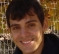## Error correcting codesStarted by 3 years ago3 replieslatest reply 3 years ago118 views

Hi All,

I would like to know if my understand as given below is correct,

Error correcting codes can be classified into

1) Block codes

Block codes are memory less

2) convolution codes

convolution codes can be further of the following,

1) systematic

2) non-systematic

3) recursive

4) non recursive

5) Multiple combinations of 1,2 with 3,4. E.g. systematic and recursive etc.

Let me know if my understanding, broadly, is correct ...

[ - ]Yes,

I would add that Block codes can be linear, systematic and cyclic.

I don't know if non-linear block code are used in practice.

[ - ]Kind of, but some of those terms, like "block code" aren't universally defined.  e.g., a "block code" can be a code with a finite-length or fixed-length codeword, like a Reed-Solomon or other algebraic code, but a "block code" can also be implemented with, and often is implemented with, a convolutional code.

Likewise block codes can be systematic, have recursive and non-recursive elements, etc.

I don't know what you mean by "memory less".

It is a broad topic with many paths.

[ - ]